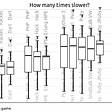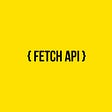# DS with JS — Hash Tables— I

## Data Structures with JavaScript — Chapter Four — Hash tables — I

Hash tables is a data structure in which every value is associated with a key and we can find that key using hash function.

# Prerequisites

Before proceeding further, make sure you have gone through both of these posts first because we will use Linked-Lists in Hash Tables — Separate Chaining Method.

❗️All the code snippets you will see below are just Pseudo code. If you want to see working code — click here

# ⚜️ The list

1. Put
2. Remove
3. Search
4. Size
5. IsEmpty

## 🔅 Put

`put( item ) {  let key = this.computeHash( item )  return !this.table[key] ? this.table[key] = item : false;}`

## 🔅 Remove

`remove( item ) {  let key = this.computeHash( item )  return this.table[key] = undefined}`

## 🔅 Search & Size

`search( item ) {  let key = this.computeHash( item )  return this.table[key] === item}size() {  let counter = 0;  for( let i=0, len = this.table.length; i < len; i++) {   if( this.table[i] ) { counter++ }  }  return counter;}`

❗️The technique which I am using to find out size is very time consuming. This is one way but the better one will be to use a counter for size. Just increment that counter whenever we put an item in Hash table and decrement it when we delete an item. Just return that counter in the size function so that we do not have to iterate over whole Hash Table very time we call it ( Some geek pointed this on reddit thread… thank you) and in next post I will implement this.

Then why are you still using it here ?
I am still using this because you can use this piece of code for a lot of other things i.e you can use this to traverse over whole Hash Table and implement a function ( may be by some reason you want every value to be in a lowercase or uppercase) over each item which will help you do a lot of other things when you are practicing this. So, this code is just to show that this is one way to do this but as I mentioned that this is not one of the best way to this sort of work. Use pointers instead.

## 🔅 IsEmpty

`isEmpty() {  for( let i=0, len = this.table.length; i < len; i++) {   if( this.table[i] ) { return false };  }  return true}`

# It’s just the beginning with hash tables…

## Let us see the real issue with Hash Functions —

In the example above, I am using a very simple Hash Function which is —

`computeHash( string ) {  let H = this.findPrime( this.table.length )  let total = 0;  for (let i = 0; i < string.length; ++i) {    total += H * total + string.charCodeAt(i)  }  return total % this.table.length}`

⚠️ In the function above, I am using a prime number. I know a lot of you are scratching their heads that why I used this. Well, it is playing a great role here.

Want to know why do hash functions use prime numbers?

There are a lot of ways you can design your own Hash Functions but no matter how complex you make you Hash Function but there is always high possibility that they will face one issue and that is —

🤜 Hash Table Collisions 🤛

…means when Hash Function returns same hash for more than one values.

There are various solutions to this problem —

but in this post, we will go through one of major of them — Separate chaining.

# Hash Tables — Separate Chaining Method

In this method, the hash table will contain links to linked-lists and we will search and insert the item by iterating over these linked-lists.

# ⚜️ The list

1. Put
2. Remove
3. Contains
4. Size
5. IsEmpty
6. Traverse

## 🔅 Put

`put( item ) {   let key = this.computeHash( item )   let node = new Node(item)   if ( this.table[key] ) {     node.next = this.table[key]   }   this.table[key] = node}`

## 🔅 Remove

`remove( item ) {  let key = this.computeHash( item )  if( this.table[key] ) {     if( this.table[key].data === item ) {        this.table[key] = this.table[key].next     } else {       let current = this.table[key].next       let prev = this.table[key]       while( current ) {         if( current.data === item ) {           prev.next = current.next         }         prev = current         current = current.next       }     }  }}`

## 🔅 Contains

`contains( item ) {  for(let i=0; i<this.table.length; i++){    if( this.table[i] ) {      let current = this.table[i];      while( current ) {        if( current.data === item ) {          return true;        }        current = current.next;      }    }  }  return false;}`

## 🔅 Size & IsEmpty

`size( item ) {  let counter = 0  for(let i=0; i<this.table.length; i++){    if( this.table[i] ) {      let current = this.table[i]      while( current ) {        counter++        current = current.next      }    }  }  return counter}isEmpty() {  return this.size() < 1}`

## 🔅 Traverse

`traverse( fn ) {  for(let i=0; i<this.table.length; i++){    if( this.table[i] ) {      let current = this.table[i];      while( current ) {        fn( current );        current = current.next;      }    }  }}`

# That’s It

This post is the fourth instalment to its series “DS with JS”. If you haven’t gone through them click here. The rest of the hashing will be covered in the next post.

There will be more in this series. Next will be on next Thrusday Morning. Stay tuned!

## Happy Coding !!

🎧 Listening to The Wolf & The Moon… Excellent music.. Soulful…

If you like this article, please give it some claps 👏 and share it! If you do not like this post or have any type of questions regarding anything that i mentioned in this post. Feel free to ask me. Just post an issue in my “Ask Me Anything” by clicking here.

--

--

--

## More from _devblogs

Stories for Full-Stack Web developers which help them in pursuing their goals as a developer, mastering the modern web technologies and *hacking the web.

## Internationalizing and Localizing Your App, Part 3: Technical Challenges## Laravel 8— Controllers tutorial with code example 2020## Doing Deals Faster with Contract Mindmapping## Use IntelliTrace Historical Debugging to fix hard bugs.## Erlang. Safe optimization with NIF on Rust## A standalone WebAssembly VM benchmark## Gaurav Mehla

Software engineer & Web hacker. Spent 30+% of life on playing with JS

## An Introduction To NodeJS## How to use fetch in NodeJS## What Is The MERN Stack, and How Does It Work?